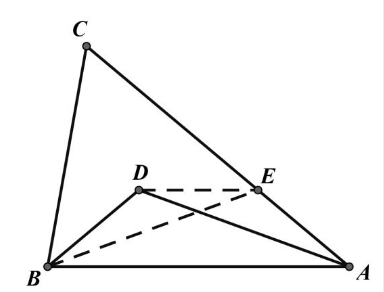【答案】

\begin{aligned} & A D=B E, B C=B E \\ & \angle C=\angle C E B=3 \angle A B E \\ & \angle C B E=3 \angle A B E \\ & \therefore \triangle B C E \text { 为等边三角形 } \\ & \angle A C B=60^{\circ} \text { 。 } \end{aligned}